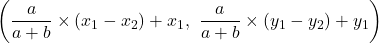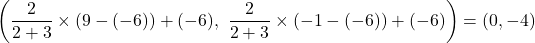## What are the coordinates of the point on the directed line segment from (-6, -6)(−6,−6) to (9, -1)(9,−1) that partitions the segment into a

Question

What are the coordinates of the point on the directed line segment from (-6, -6)(−6,−6) to (9, -1)(9,−1) that partitions the segment into a ratio of 2 to 3?

in progress 0
4 months 2021-09-05T10:55:32+00:00 1 Answers 30 views 0

(0, -4)

Step-by-step explanation:

The coordinates of the points from which the directed line segment extends = (-6, -6) to (9, -1)

The ratio the required point partitions the line = 2 to 3

The formula for finding the coordinate of a point that partitions a line AB into a ratio ‘a’ to ‘b’, where the coordinates of, A = (x₁, y₁) and B = (x₂, y₂) is given as follows;Therefore, the required point is located as follows;The coordinates of the point is (0, -4)